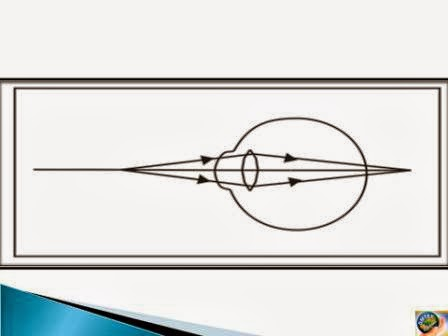## வியாழன், 2 பிப்ரவரி, 2017

### SCIENCE MARCH 2016 PAPER ONE

Q. 1. A. (1) Fill in the blanks 

(1) The modern periodic table consist of ___________ periods. (Seven)

(2) The formulae of chloride of metal M is MCl2. The metal M belongs to ___________ group. (II A)

(3) Corrosion can be prevented by using _____________ solution. (anti - rust)

(2) Find the odd one out: 

(1) Voltmeter, ammeter, galvanometer, thermometer.

Ans. Thermometer. (Thermometer is used to measure temperature. Voltmeter, ammeter and galvanometer are concerned with electrical quantities. )

(2) Loudspeaker, bar magnet, electric motor, microphone.

Ans. Barmagnet. (Bar magnet does not need electrical energy to function. But all others need electrical energy to function. )

(B) Choose the correct alternative and rewrite the following: 

(1) Reaction of ion nails with copper sulphate solution is an example of:
(a) Combination reaction
(b) Decomposition reaction
(c) Displacement reaction.
(d) Double displacement reaction .

(2) Dilute NaOH can be tested with _________
(a) red litmus paper
(b) blue litmus paper
(c) lime water
(d) Na2CO3.

(3) In series combination which remains constant?
(a) Voltage
(b) Current
(c) Both current and voltage
(d) Both are variables.

(4) An object of 10 cm is placed in front of a plane mirror. The height of image will be ____________
(a) 5 cm
(b) 15 cm
(c) 20 cm
(d) 10 cm

(5) When a ray of light travels from air to glass slab and strikes the surface of separation at 900, then it ____________
(a) bends towards normal
(b) bends away from normal
(c) passes unbent
(d) passes in zigzag way.

Q. II. Answer the following questions. (any five)  [5 x 2 = 10]

(1) Define the following:

(2) What major harm is done to the human beings by air pollution?

(3) Calculate the focal length of a corrective lens having power +2D.

(4) Write two applications of Sodium bicarbonate (baking soda)

(5) A simple microscope is used by watch repairers. Give reason.

(6) Draw neat and labelled diagram of pH scale.

Q. III. Answer the following questions. (any five): [5 x 3 = 15]

(1) What are the main features of Mendeleev’s periodic table?

(2) Define ‘redox reaction’. Give one example.

(3) Given below is a diagram showing a defect of human eye. Study it and answer the following questions.(a) Name the defect shown in the figure.
(b) Give reason for this defect of eye in human being.
(c) Name the type of lens used to correct the eye defect.

(4) What is refraction of light? How is it related to refractive index?

(5) Explain how spectrum is formed.

(6) Suggest measures in the following situations:
(a) To avoid noise pollution in classroom.
(b) To minimize electricity consumption at home.
(c) Bursting fire crackers in festivals and processions.

Q. IV. Answer any one of the following questions: 

(1) Find the expression for the resistors connected in series and write the two characteristics of it. (Draw figure)

(2) Explain ‘Electric motor’ with the help of the following points:
(a) Draw figure.
(b) Principle of Electric motor.
(c) Name any four appliances where electric motor is used.